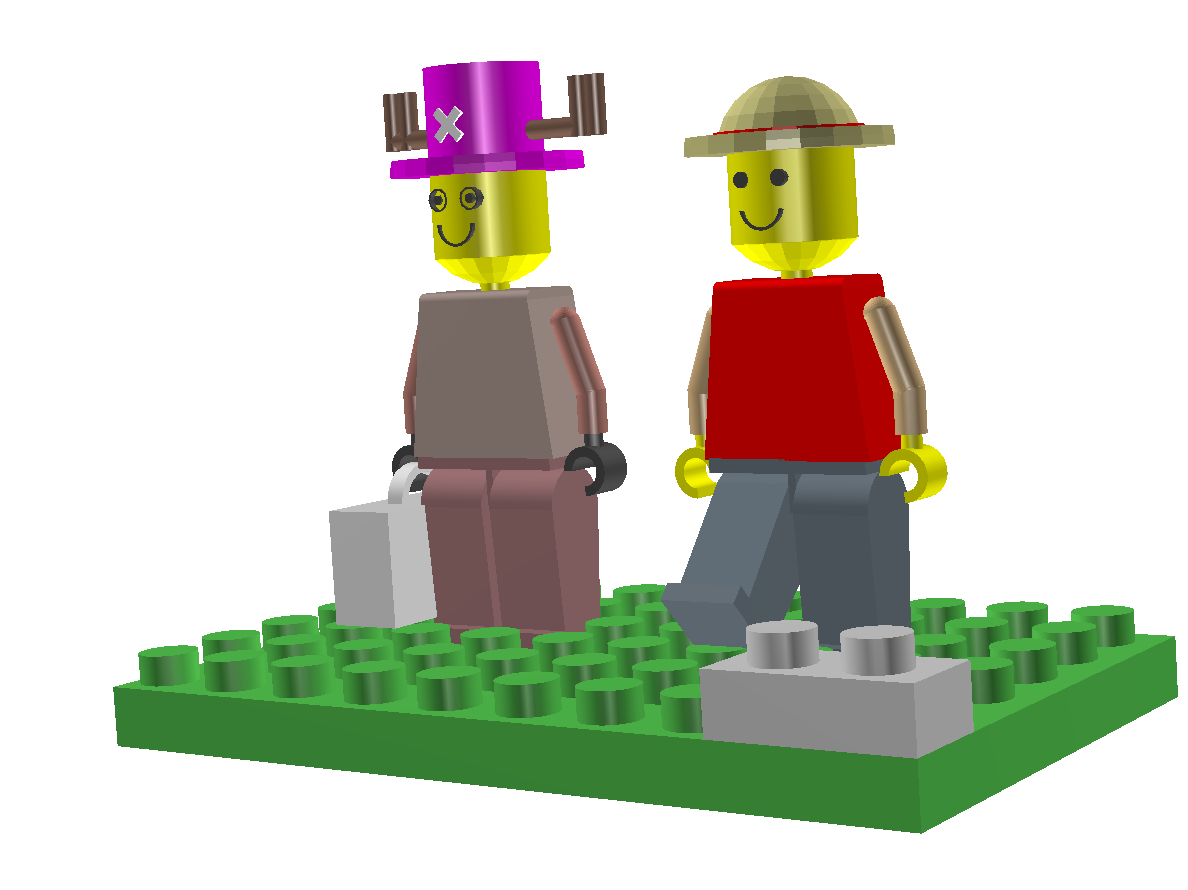ROOT   Reference Guidelego.C File Reference

## Detailed Description

Drawing a figure, made of lego block, using ROOT geometry class.

Reviewed by Sunman Kim (sunma.nosp@m.n98@.nosp@m.hanma.nosp@m.il.n.nosp@m.et) Supervisor: Prof. Inkyu Park (icpar.nosp@m.k@ph.nosp@m.ysics.nosp@m..uos.nosp@m..ac.k.nosp@m.r)

How to run: .x lego.C in ROOT terminal, then use OpenGL

This macro was created for the evaluation of Computational Physics course in 2006. We thank to Prof. Inkyu Park for his special lecture on ROOT and to all of ROOT team#include "TSystem.h"
#include "TGeoManager.h"
void lego()
{
TGeoManager *geom = new TGeoManager("geom","My first 3D geometry");
TGeoMaterial *vacuum=new TGeoMaterial("vacuum",0,0,0);
TGeoMaterial *Fe=new TGeoMaterial("Fe",55.845,26,7.87);
TGeoMedium *Air=new TGeoMedium("Vacuum",0,vacuum);
TGeoMedium *Iron=new TGeoMedium("Iron",1,Fe);
// create volume
TGeoVolume *top=geom->MakeBox("top",Air,100,100,100);
geom->SetTopVolume(top);
geom->SetTopVisible(0);
// If you want to see the boundary, please input the number, 1 instead of 0.
// Like this, geom->SetTopVisible(1);
//----------------------------------------------------------------------
TGeoVolume *ha1=geom->MakeSphere("ha1",Iron,0,10,80,90,0,360);
ha1->SetLineColor(41);
TGeoVolume *ha2=geom->MakeSphere("ha2",Iron,0,7,90,180,0,360);
ha2->SetLineColor(41);
TGeoVolume *ha3=geom->MakeSphere("ha3",Iron,0,7.3,80,90,0,360);
ha3->SetLineColor(2);
TGeoVolume *h1=geom->MakeTubs("h1",Iron,0,6,4.5,0,0);
TGeoVolume *h2=geom->MakeSphere("h2",Iron,0,7.5,0,52.5,0,360);
h2->SetLineColor(5);
TGeoVolume *h3=geom->MakeSphere("h3",Iron,0,7.5,0,52.5,0,360);
h3->SetLineColor(5);
TGeoVolume *h4=geom->MakeTubs("h4",Iron,2.5,3.5,1.5,0,0);
h4->SetLineColor(5);
TGeoVolume *t1_1=geom->MakeTubs("t1_1",Iron,0,0.8,1,0,360);
t1_1->SetLineColor(12);
TGeoVolume *t2_1=geom->MakeTubs("t2_1",Iron,0,0.8,1,0,360);
t2_1->SetLineColor(12);
TGeoVolume *fb1=geom->MakeTubs("fb1",Iron,2,2.3,1,100,260);
fb1->SetLineColor(12);
TGeoVolume *m1=geom->MakeBox("m1",Iron,7,8,4);
m1->SetLineColor(2);
TGeoVolume *m2=geom->MakeTubs("m2",Iron,0,1,7,90,180);
m2->SetLineColor(2);
TGeoVolume *m3=geom->MakeTubs("m3",Iron,0,1,7,0,90);
m3->SetLineColor(2);
TGeoVolume *m4=geom->MakeBox("m4",Iron,3,7,0.5);
m4->SetLineColor(2);
TGeoVolume *m5=geom->MakeTubs("m5",Iron,0,1.5,1.2,0,0);
m5->SetLineColor(5);
TGeoVolume *m6=geom->MakeTrd2("m6",Iron,4,4,0,2,8);
m6->SetLineColor(2);
TGeoVolume *m7=geom->MakeTrd2("m7",Iron,4,4,0,2,8);
m7->SetLineColor(2);
TGeoVolume *md1=geom->MakeBox("md1",Iron,4,8.5,0.7);
md1->SetLineColor(37);
TGeoVolume *md2=geom->MakeBox("md2",Iron,3,0.4,2);
md2->SetLineColor(37);
TGeoVolume *d1=geom->MakeTrd2("d1",Iron,3,4,4,4,7);
d1->SetLineColor(37);
TGeoVolume *d2=geom->MakeTrd2("d2",Iron,3,4,4,4,7);
d2->SetLineColor(37);
TGeoVolume *d3=geom->MakeTubs("d3",Iron,0,4,3.98,0,180);
d3->SetLineColor(37);
TGeoVolume *d4=geom->MakeTubs("d4",Iron,0,4,3.98,0,180);
d4->SetLineColor(37);
TGeoVolume *d5=geom->MakeBox("d5",Iron,4,4,1);
d5->SetLineColor(37);
TGeoVolume *d6=geom->MakeBox("d6",Iron,4,4,1);
d6->SetLineColor(37);
TGeoVolume *a1=geom->MakeTubs("a1",Iron,0,1.5,4,0,0);
a1->SetLineColor(1);
TGeoVolume *a2=geom->MakeSphere("a2",Iron,0,1.48,0,180,0,200);
a2->SetLineColor(1);
TGeoVolume *a3=geom->MakeTubs("a3",Iron,0,1.5,2.2,0,0);
a3->SetLineColor(1);
TGeoVolume *a4=geom->MakeTubs("a4",Iron,0,1,1,0,0);
a4->SetLineColor(5);
TGeoVolume *a5=geom->MakeTubs("a5",Iron,1.5,2.5,2,0,270);
a5->SetLineColor(5);
TGeoVolume *a1_1=geom->MakeTubs("a1_1",Iron,0,1.5,4,0,0);
a1_1->SetLineColor(1);
TGeoVolume *a2_1=geom->MakeSphere("a2_1",Iron,0,1.48,0,180,0,200);
a2_1->SetLineColor(1);
TGeoVolume *a3_1=geom->MakeTubs("a3_1",Iron,0,1.5,2.2,0,0);
a3_1->SetLineColor(1);
TGeoVolume *a4_1=geom->MakeTubs("a4_1",Iron,0,1,1,0,0);
a4_1->SetLineColor(5);
a5=geom->MakeTubs("a5_1",Iron,1.5,2.5,2,0,270);
a5->SetLineColor(5);
//**********************************NO,2******************
TGeoVolume *ha_1=geom->MakeSphere("ha_1",Iron,0,10,80,90,0,360);
ha_1->SetLineColor(6);
TGeoVolume *ha_2=geom->MakeTubs("ha_2",Iron,0,6,5,0,0);
ha_2->SetLineColor(6);
TGeoVolume *ha_3=geom->MakeTubs("ha_3",Iron,0,1,12,0,0);
ha_3->SetLineColor(28);
TGeoVolume *ha_4=geom->MakeTubs("ha_4",Iron,0,1,3,0,0);
ha_4->SetLineColor(28);
TGeoVolume *ha_5=geom->MakeTubs("ha_5",Iron,0,1,3,0,0);
ha_5->SetLineColor(28);
TGeoVolume *ha_6=geom->MakeTubs("ha_6",Iron,0,1,3,0,0);
ha_6->SetLineColor(28);
TGeoVolume *ha_7=geom->MakeTubs("ha_7",Iron,0,1,3,0,0);
ha_7->SetLineColor(28);
TGeoVolume *ha_8=geom->MakeBox("ha_8",Iron,2,0.5,2);
ha_8->SetLineColor(19);
TGeoVolume *ha_9=geom->MakeBox("ha_9",Iron,2,0.5,2);
ha_9->SetLineColor(19);
TGeoVolume *h_1=geom->MakeTubs("h_1",Iron,0,6,4.5,0,0);
h_1->SetLineColor(5);
TGeoVolume *h_2=geom->MakeSphere("h_2",Iron,0,7.5,0,52.5,0,360);
h_2->SetLineColor(5);
TGeoVolume *h_3=geom->MakeSphere("h_3",Iron,0,7.5,0,52.5,0,360);
h_3->SetLineColor(5);
TGeoVolume *h_4=geom->MakeTubs("h_4",Iron,2.5,3.5,1.5,0,0);
h_4->SetLineColor(5);
TGeoVolume *fa1=geom->MakeTubs("fa1",Iron,0,0.5,1,0,360);
fa1->SetLineColor(12);
TGeoVolume *fa2=geom->MakeTubs("fa2",Iron,0,0.5,1,0,360);
fa2->SetLineColor(12);
TGeoVolume *fa1_1=geom->MakeTubs("fa1_1",Iron,1,1.2,1,0,360);
fa1_1->SetLineColor(12);
TGeoVolume *fa2_1=geom->MakeTubs("fa2_1",Iron,1,1.2,1,0,360);
fa2_1->SetLineColor(12);
TGeoVolume *fa3=geom->MakeTubs("fa3",Iron,2,2.3,1,90,270);
fa3->SetLineColor(12);
TGeoVolume *m_1=geom->MakeBox("m_1",Iron,7,8,4);
m_1->SetLineColor(25);
TGeoVolume *m_2=geom->MakeTubs("m_2",Iron,0,1,7,90,180);
m_2->SetLineColor(25);
TGeoVolume *m_3=geom->MakeTubs("m_3",Iron,0,1,7,0,90);
m_3->SetLineColor(25);
TGeoVolume *m_4=geom->MakeBox("m_4",Iron,3,7,0.5);
m_4->SetLineColor(25);
TGeoVolume *m_5=geom->MakeTubs("m_5",Iron,0,1.5,1.2,0,0);
m_5->SetLineColor(5);
TGeoVolume *m_6=geom->MakeTrd2("m_6",Iron,4,4,0,2,8);
m_6->SetLineColor(25);
TGeoVolume *m_7=geom->MakeTrd2("m_7",Iron,4,4,0,2,8);
m_7->SetLineColor(25);
TGeoVolume *md_1=geom->MakeBox("md_1",Iron,4,8.5,0.7);
md_1->SetLineColor(48);
TGeoVolume *md_2=geom->MakeBox("md_2",Iron,3,0.4,2);
md_2->SetLineColor(48);
TGeoVolume *d_1=geom->MakeTrd2("d_1",Iron,3,4,4,4,7);
d_1->SetLineColor(48);
TGeoVolume *d_2=geom->MakeTrd2("d_2",Iron,3,4,4,4,7);
d_2->SetLineColor(48);
TGeoVolume *d_3=geom->MakeTubs("d_3",Iron,0,4,3.98,0,180);
d_3->SetLineColor(48);
TGeoVolume *d_4=geom->MakeTubs("d_4",Iron,0,4,3.98,0,180);
d_4->SetLineColor(48);
TGeoVolume *d_5=geom->MakeBox("d_5",Iron,4,4,1);
d_5->SetLineColor(48);
TGeoVolume *d_6=geom->MakeBox("d_6",Iron,4,4,1);
d_6->SetLineColor(48);
TGeoVolume *a_1=geom->MakeTubs("a_1",Iron,0,1.5,4,0,0);
a_1->SetLineColor(45);
TGeoVolume *a_2=geom->MakeSphere("a_2",Iron,0,1.48,0,180,0,200);
a_2->SetLineColor(45);
TGeoVolume *a_3=geom->MakeTubs("a_3",Iron,0,1.5,2.2,0,0);
a_3->SetLineColor(45);
TGeoVolume *a_4=geom->MakeTubs("a_4",Iron,0,1,1,0,0);
a_4->SetLineColor(12);
TGeoVolume *a_5=geom->MakeTubs("a_5",Iron,1.5,2.5,2,0,270);
a_5->SetLineColor(12);
TGeoVolume *Aa1=geom->MakeTubs("Aa1",Iron,0,1.5,4,0,0);
Aa1->SetLineColor(45);
TGeoVolume *Aa2=geom->MakeSphere("Aa2",Iron,0,1.48,0,180,0,200);
Aa2->SetLineColor(45);
TGeoVolume *Aa3=geom->MakeTubs("Aa3",Iron,0,1.5,2.2,0,0);
Aa3->SetLineColor(45);
TGeoVolume *Aa4=geom->MakeTubs("Aa4",Iron,0,1,1,0,0);
Aa4->SetLineColor(12);
TGeoVolume *Aa5=geom->MakeTubs("Aa5",Iron,1.5,2.5,2,0,270);
Aa5->SetLineColor(12);
TGeoVolume *bag1=geom->MakeBox("bag1",Iron,10,4,6);
bag1->SetLineColor(19);
TGeoVolume *bag2=geom->MakeTubs("bag2",Iron,3,4,1,180,360);
bag2->SetLineColor(19);
TGeoVolume *well=geom->MakeBox("well",Iron,5,10,3);
well->SetLineColor(18);
TGeoVolume *K5=geom->MakeTubs("K5",Iron,0,3,3,0,0);
K5->SetLineColor(18);
TGeoVolume *K4=geom->MakeTubs("K4",Iron,0,3,3,0,0);
K4->SetLineColor(18);
//==============Board=========
char nB;
int Z=0,Y=0;
TGeoVolume *bo1;
while(Y<6){
while(Z<10){
sprintf(nB,"B%d_Y%d",Z,Y);
bo1=geom->MakeTubs(nB,Iron,0,3,3,0,0);
bo1->SetLineColor(8);
Z++;
}
Y++; Z=0;
}
TGeoVolume *bo2=geom->MakeBox("bo2",Iron,27,45,3);
bo2->SetLineColor(8);
top->SetVisibility(0);
geom->CloseGeometry();
top->Draw("ogl");
}
virtual void SetLineColor(Color_t lcolor)
Set the line color.
Definition: TAttLine.h:40
Class describing rotation + translation.
Definition: TGeoMatrix.h:292
The manager class for any TGeo geometry.
Definition: TGeoManager.h:45
void CloseGeometry(Option_t *option="d")
Closing geometry implies checking the geometry validity, fixing shapes with negative parameters (run-...
TGeoVolume * MakeTrd2(const char *name, TGeoMedium *medium, Double_t dx1, Double_t dx2, Double_t dy1, Double_t dy2, Double_t dz)
Make in one step a volume pointing to a TGeoTrd2 shape with given medium.
TGeoVolume * MakeBox(const char *name, TGeoMedium *medium, Double_t dx, Double_t dy, Double_t dz)
Make in one step a volume pointing to a box shape with given medium.
TGeoVolume * MakeSphere(const char *name, TGeoMedium *medium, Double_t rmin, Double_t rmax, Double_t themin=0, Double_t themax=180, Double_t phimin=0, Double_t phimax=360)
Make in one step a volume pointing to a sphere shape with given medium.
void SetTopVolume(TGeoVolume *vol)
Set the top volume and corresponding node as starting point of the geometry.
void SetTopVisible(Bool_t vis=kTRUE)
make top volume visible on screen
TGeoVolume * MakeTubs(const char *name, TGeoMedium *medium, Double_t rmin, Double_t rmax, Double_t dz, Double_t phi1, Double_t phi2)
Make in one step a volume pointing to a tube segment shape with given medium.
Base class describing materials.
Definition: TGeoMaterial.h:36
Media are used to store properties related to tracking and which are useful only when using geometry ...
Definition: TGeoMedium.h:24
Class describing rotations.
Definition: TGeoMatrix.h:175
TGeoVolume, TGeoVolumeMulti, TGeoVolumeAssembly are the volume classes.
Definition: TGeoVolume.h:49
virtual void AddNodeOverlap(TGeoVolume *vol, Int_t copy_no, TGeoMatrix *mat=nullptr, Option_t *option="")
Add a TGeoNode to the list of nodes.
Definition: TGeoVolume.cxx:997
virtual void SetVisibility(Bool_t vis=kTRUE)
set visibility of this volume
virtual void Draw(Option_t *option="")
draw top volume according to option
virtual void SetLineColor(Color_t lcolor)
Set the line color.
void lego()
Definition: lego.C:31
TH1F * h1
Definition: legend1.C:5
static constexpr double m3
static constexpr double m2

Definition in file lego.C.